This article shows how to estimate and visualize a two-dimensional cumulative distribution function (CDF) in SAS. SAS has built-in support for this computation. Although the bivariate CDF is not used as much as the univariate CDF, the bivariate version is still a useful tool in understanding the probable values of a random variate for a two-dimensional distribution.

Recall that the univariate CDF at the value x is the probability that a random observation from a distribution is less than x. In symbols, if X is a random variable, then the distribution function is $F_X(x) = P(X\leq x)$. The two-dimensional CDF is similar, but it gives the probability that two random variables are both less than specified values. If X and Y are random variables, the bivariate CDF for the joint distribution is given by $F_{XY}(x,y) = P(X \leq x ~\mbox{and}~ Y \leq y)$.

### Univariate estimates of the CDF

Let's start with a one-dimensional example before exploring the topic in higher dimensions. For univariate probability distributions, statisticians use both the density function and the cumulative distribution function. They each contain the same information, and if you know one you can find the other. The cumulative distribution (CDF) of a continuous distribution function is the integral of the probability density function (PDF); the density function is the derivative of the cumulative distribution.

In terms of data analysis, a histogram is an estimate of a density function. So is a kernel density estimate. Consequently, there are three popular methods for estimating a CDF from data:

Let's compare the first and last methods to estimate the CDF. For data, use the MPG_City variable from the Sashelp.Cars data.

/* Use PROC UNIVARIATE to compute the empirical CDF from data */ proc univariate data=sashelp.Cars; /* use the FREQ option to get a table */ var MPG_City; cdfplot MPG_City; ods select CDFPlot; ods output CDFPlot=UNIOut; run;   /* Use PROC KDE to estimate the CDF from a kernel density estimate */ proc kde data=sashelp.Cars; univar MPG_City / CDF out=KDEOut; run;   /* combine the estimates and plot on the same graph */ data All; set UNIOut(rename=(ECDFY=ECDF ECDFX=X)) KDEOut(rename=(distribution=kerCDF Value=X)); ECDF = ECDF / 100; /* convert from percent to proportion */ keep X ECDF kerCDF; run;   title "Estimates of CDF"; proc sgplot data=All; label X = "MPG_City"; series x=X y=ECDF / legendlabel="Empirical CDF"; series x=X y=kerCDF / legendlabel="Kernel CDF"; yaxis label="Cumulative Distribution" grid; xaxis values=(5 to 60 by 5) grid valueshint; run;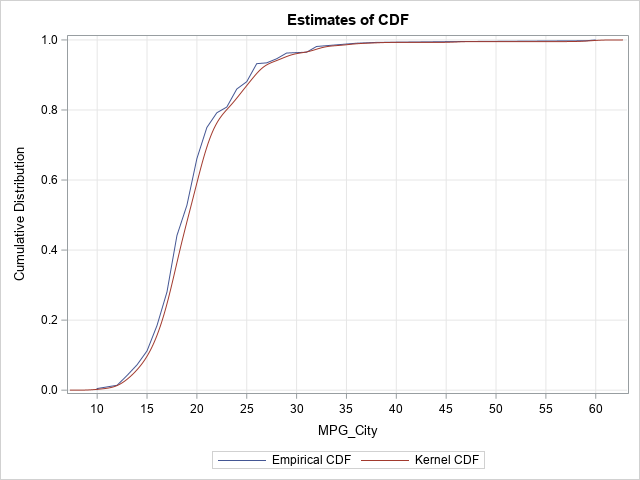The two estimates are very close. The empirical CDF is more "jagged," whereas the estimate from the kernel density is smoother. You could use either curve to estimate quantiles or probabilities.

### Bivariate estimates of the CDF

Now onto the bivariate case. For bivariate data, you cannot use PROC UNIVARIATE (obviously, from the name!), but you can still use PROC KDE. Instead of using the UNIVAR statement, you can use the BIVAR statement to compute a bivariate KDE. Let's look at the joint distribution of the MPG_City and Weight variables in the Sashelp.Cars data:

title; proc sgplot data=sashelp.Cars; scatter x=MPG_City y=Weight; run;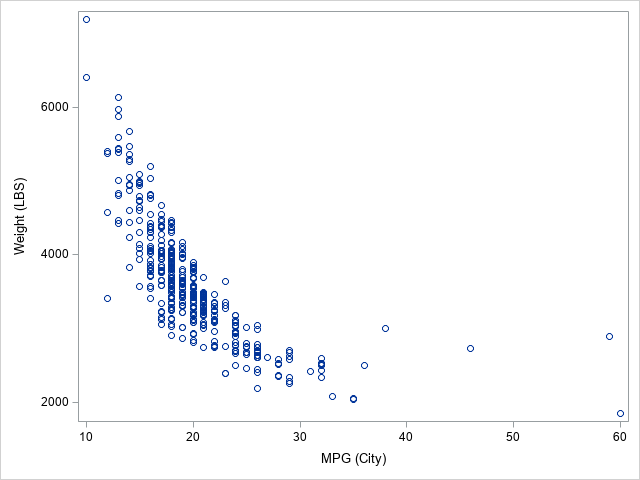The scatter plot shows that the density of these points is concentrated near the lower-left corner of the plot. You can use PROC KDE to visualize the density. The PLOTS= option can create a contour plot and a two-dimensional histogram, as follows:

title "2-D Histogram"; proc kde data=sashelp.Cars; bivar MPG_City Weight / plots=(Contour Histogram) CDF out=KDEOut(rename=(Distribution=kerCDF Value1=X Value2=Y)); run;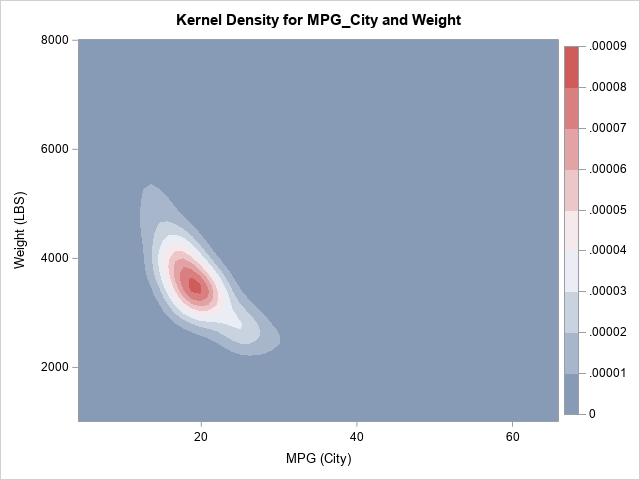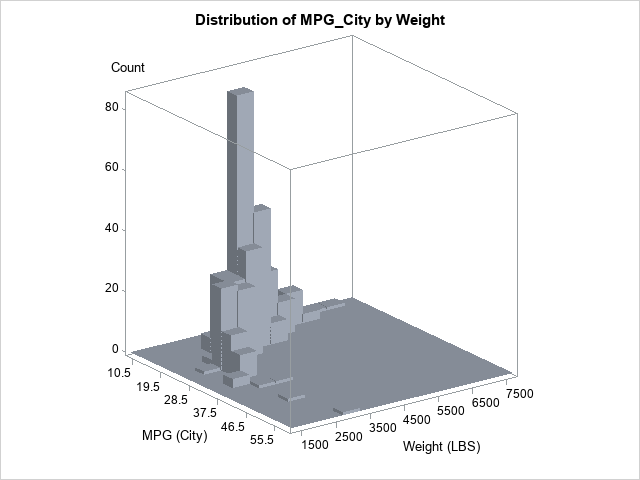The OUT= option writes a SAS data set that contains the estimates for the density; the CDF option adds the cumulative distribution estimates. The column names are Value1 (for the first variable on the BIVAR statement), Value2 (for the second variable), and Distribution (for the CDF). I have renamed the variables to X, Y, and CDF, but that is not required. You can plot use a heat map (or a contour plot) to visualize the bivariate CDF, as follows:

title "Estimate of 2-D CDF"; proc sgplot data=KDEOut; label X="MPG_City" Y="Weight" kerCDF="Kernel CDF"; heatmapparm x=X y=Y colorresponse=kerCDF; run;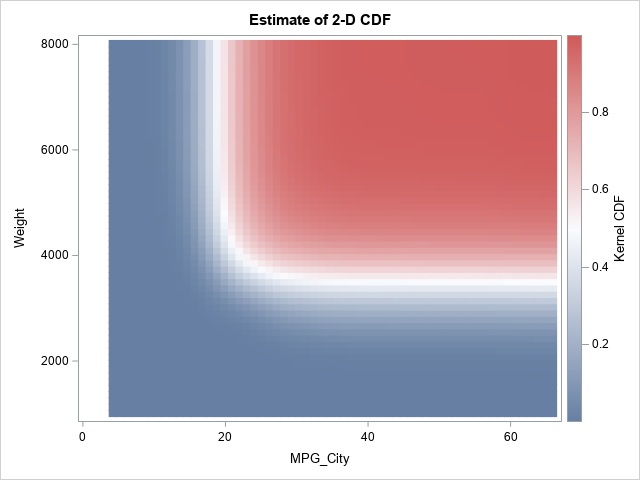### The CDF of bivariate normal data

The CDF is not used very often for bivariate data because the density function shows more details. In some sense, all CDFs look similar. In the previous example, the two variables are negatively correlated and are not linearly related. For comparison, the following program uses the RANDNORMAL function in SAS/IML to generate bivariate normal data that has a positive correlation of 0.6. (I have previously shown the empirical CDF for this data, and I have also drawn the graph of the bivariate CDF for the population.) The calls to PROC KDE and PROC SGPLOT are the same as for the previous example:

proc iml; call randseed(123456); N = 1e5; /* sample size */ rho = 0.6; Sigma = ( 1 || rho)// /* correlation matrix */ (rho|| 1 ); mean = {0 0}; Z = randnormal(N, mean, Sigma); /* sample from MVN(0, Sigma) */ create BivNorm from Z[c={X Y}]; append from Z; close; quit;   proc kde data=BivNorm; bivar X Y / plots=NONE CDF out=KDEOut(rename=(distribution=kerCDF Value1=X Value2=Y)); run;   title "Estimate of 2-D Normal CDF"; proc sgplot data=KDEOut aspect=1; label kerCDF="Kernel CDF"; heatmapparm x=X y=Y colorresponse=kerCDF; run;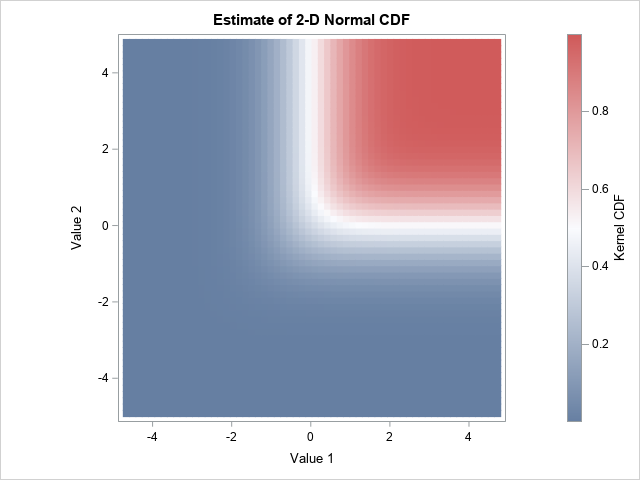If you compare the bivariate CDF for the Cars data to the CDF for the bivariate normal data, you can see differences in the range of the red region, but the overall impression is the same. The CDF is near 0 in the lower-left corner, near 1 in the upper-right corner, and is approximately 0.5 along an L-shaped curve near the middle of the data. This is the basic shape of a generic 2-D CDF, just as an S-shape or sigmoid-shaped curve is the basic shape of a generic 1-D CDF.

### Summary

You can use the CDF option on the BIVAR statement in PROC KDE to obtain an estimate for a bivariate CDF. The estimate is based on a kernel density estimate of the data. You can use the HEATMAPPARM statement in PROC SGPLOT to visualize the CDF surface. If you prefer a contour plot, you can use the graph template language (GTL) to create a contour plot.

ShareDistinguished Researcher in Computational Statistics

Rick Wicklin, PhD, is a distinguished researcher in computational statistics at SAS and is a principal developer of SAS/IML software. His areas of expertise include computational statistics, simulation, statistical graphics, and modern methods in statistical data analysis. Rick is author of the books Statistical Programming with SAS/IML Software and Simulating Data with SAS.

1.You are amazing.

2.Rick,
Using PROC G3D could better display the CDF graph for bivariate .

proc g3d data=KDEout;
plot x*y=kerCDF;
run;

•Thanks. A 3-D surface plot looks cool, but for most applications, I recommend a heat map or contour plot. I find that it is easier to estimate the values of the function at specific (x,y) locations, and you never have to worry about choosing a tilt/rotation because the entire surface is visible.

3.Rick,
Or using GTL to get this 3D CDF surface.

proc template;
define statgraph SurfacePlot;
BeginGraph;
layout overlay3d;
surfaceplotparm x=x y=y z=kerCDF / surfacetype=fill colormodel=(green yellow red) colorresponse=kerCDF ;
endlayout;
EndGraph;
end;
run;

proc sgrender data=KDEout template=SurfacePlot;run;

4.wudneh ketema on

Hi Dr Rick
How are you?
I have a bit time when I read your work, I really wonderful.
If you have please attach regarding to negative variance estimate in mixed model by sas iml example ?

•There is a SAS Usage note about negative variance estimates in mixed models. In practice, researchers treat the variable as having 0 variance and remove that effect from the model.

5.wudneh ketema on

thanks a lot

6.wudneh ketema on

you may have simple example for sas iml for mixed model

•I do not have SAS IML code for fitting mixed models. I use PROC MIXED or GLIMMIX.

7.Bruno Mario Cesama on

Dear Rick,
I am not able to find in SAS 9.4 (IML 15.1) a function that returns the probability of a bivariate Student's t such as the function "pmvt" of the free R language.
In any case it is possible to obtain the results of the pmvt in SAS (IML)?.
Many thanks for your attention and help.
Best
Bruno

•There is not a built-in function for the CDF of the bivariate t distribution.